​一篇文章总结一下Python库中关于时间的常见操作

## time包

``import time``

### 时间戳

`1970年1月1日00:00:00`标准时区诞生到现在时间一共过了多少秒。

``````
timestamp = time.time()  # type:float
print(timestamp, type(timestamp))``````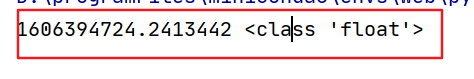### 睡眠

``````
# 睡眠 1秒
time.sleep(1)``````

### 本地时区

``````
t = time.localtime()  # type:time.struct_time
print(t, type(t))``````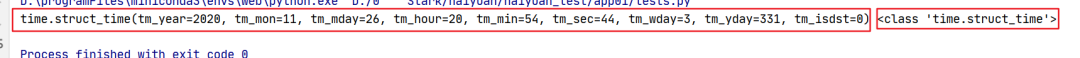`localtime`还能接收一个时间戳参数。

``````# 将时间戳转换成 struct_time 对象
t = time.localtime(1606395685.1878598)  # type:time.struct_time
print(t, type(t))``````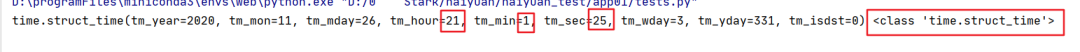### 简单的时间格式

``````
t = time.ctime()  # type:str
print(t, type(t))``````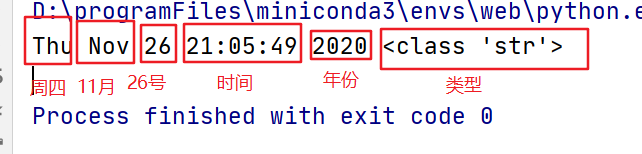``````
t = time.ctime(1606395685.1878598)  # type:str
print(t, type(t))``````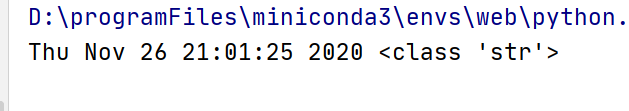### 日期格式 -> 字符串(strftime)

``````t = time.localtime()  # type:time.struct_time
t_str = time.strftime("%Y-%m-%d", t)  # type:str
print(t_str, type(t_str))``````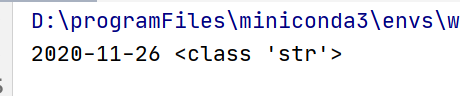### 字符串日期 -> 日期(strptime)

``````t_str = "2020-11-02"
t_time = time.strptime(t_str, "%Y-%m-%d")  # type:time.struct_time
print(t_time, type(t_time))``````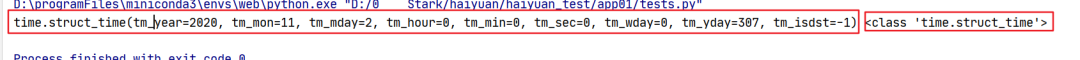### 格式化补充

%Y 完整的年份
%m 月份（01 - 12）
%d 一个月中的第几天（01 - 31
%H 一天中的第几个小时（24小时制，00 - 23）
%M 分钟数（00 - 59）
%S 秒（01 - 61）

``https://www.runoob.com/python/python-date-time.html``

## datetime包

``from datetime import datetime``

### datetime.today()

``````t = datetime.today()  # type:datetime
print(t, type(t))
print(t.year)  # 年份
print(t.month)  # 月份``````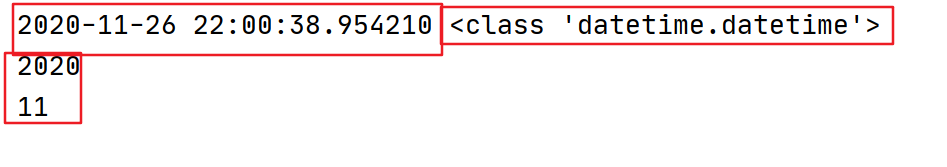### datetime.now()

`datetime.today()`基本一样,返回的是本地时间

``````
t = datetime.now()  # type:datetime
print(t,type(t))``````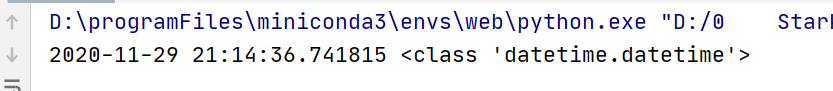### datetime.utcnow()

`utcnow`返回的是标准(UTC)时间,上述俩返回的都是本地时间,我们是东八区

``````
t = datetime.now()
print("东八区时间:", t)
t = datetime.utcnow()  # type:datetime
print("UTC时间:", t)``````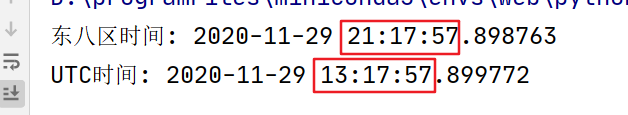### 时间戳转datetime

``````
# 时间戳
timestamp = time.time()
print(f"timestamp:{timestamp},type:{type(timestamp)}")
# 时间戳转datetime
t = datetime.fromtimestamp(timestamp)
print(f"t:{t},type:{type(t)}")``````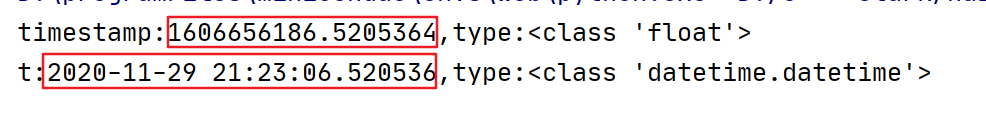### datetime -> 字符串日期(strftime)

``````from datetime import datetime

t = datetime.now()
str_datetime = t.strftime("%Y-%m-%d %H:%M:%S")
print(f"字符串日期:{str_datetime},type:{type(str_datetime)}")``````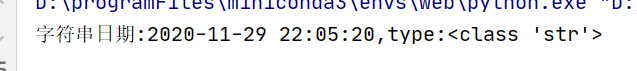### 字符串日期 -> datetime(strptime)

``````from datetime import datetime

str_datetime = "2020-11-29 22:05:20"
t = datetime.strptime(str_datetime, "%Y-%m-%d %H:%M:%S")
print(f"t:{t},type:{type(t)}")``````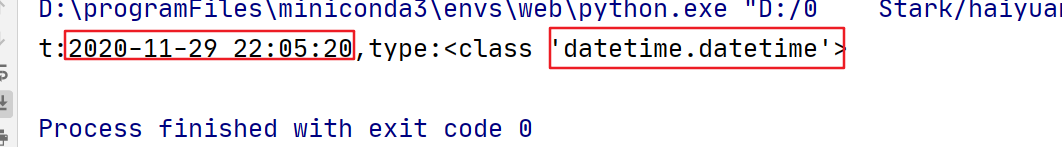### 时间加减

``````
from datetime import datetime
import datetime as idatetime

t = datetime.now()
print(f"当前时间:{t}")
three_day = t + idatetime.timedelta(days=3)
print(f"三天后时间:{three_day}")``````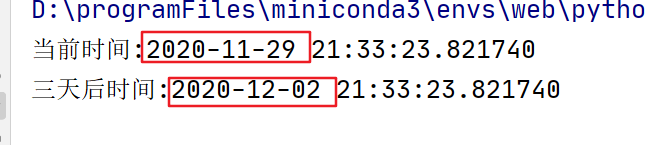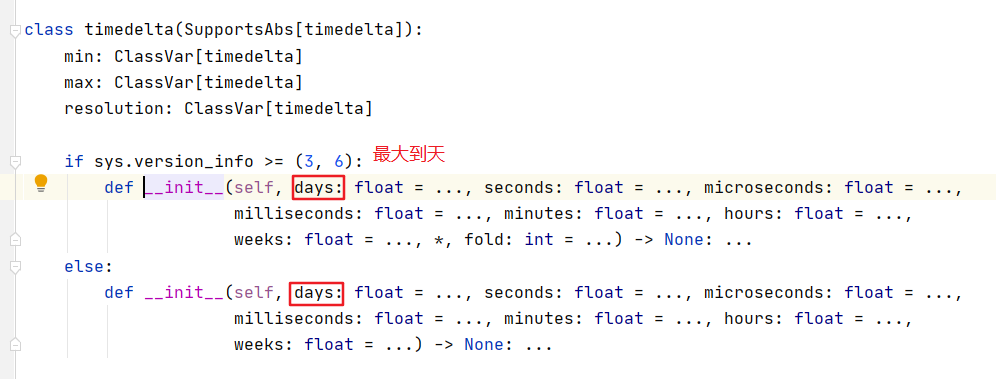datetime时间自由加减

``pip install python-dateutil``

``````
from datetime import datetime
from dateutil.relativedelta import relativedelta

t = datetime.now()
print(f"当前时间:{t}")
three_time = t + relativedelta(months=3)
print(f"三个月后时间:{three_time}")
one_year = t+relativedelta(years=1)
print(f"一年后时间:{one_year}")
up_year = t+relativedelta(years=-1)
print(f"去年这个时间:{up_year}")``````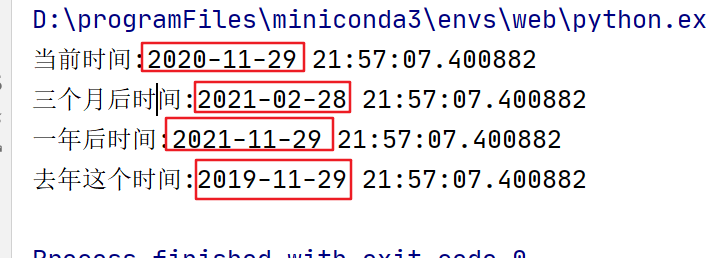## 总结

**-----**------**-----**---**** End **-----**--------**-----**-****

• ## 手把手教你用Python打造一个语音合成系统浅梦一笑
5个月前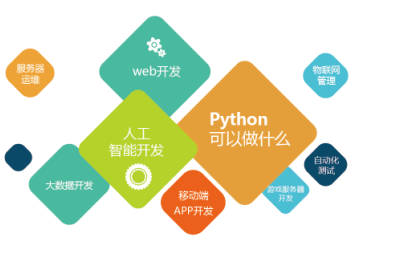blmius
1年前
MySQL:[Err] 1292 - Incorrect datetime value: ‘0000-00-00 00:00:00‘ for column ‘CREATE_TIME‘ at row 1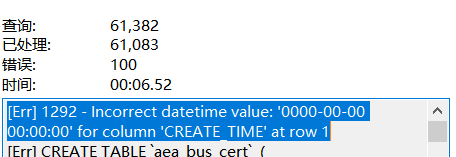Karen110
1年前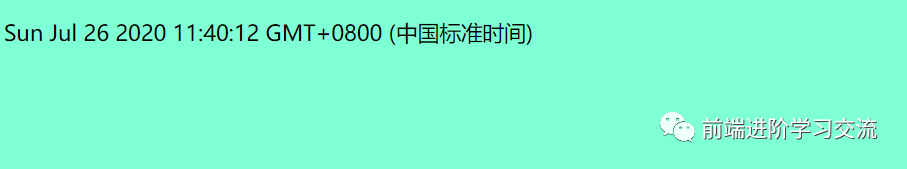Stella981
1年前
Django之Django模板
1、问：html页面从数据库中读出DateTimeField字段时，显示的时间格式和数据库中存放的格式不一致，比如数据库字段内容为2012082616:00:00，但是页面显示的却是Aug.26,2012,4p.m.答：为了页面和数据库中显示一致，需要在页面格式化时间，需要添加<td{{dayrecord.p\_time|date:Stella981
1年前
Python之time模块的时间戳、时间字符串格式化与转换
Python处理时间和时间戳的内置模块就有time，和datetime两个，本文先说time模块。关于时间戳的几个概念时间戳，根据1970年1月1日00:00:00开始按秒计算的偏移量。时间元组（struct_time），包含9个元素。 time.struct_time(tm_yStella981
1年前
QClub 太原，2014年11月15日 Global Day of Coderetreat
QClub太原，2014年11月15日GlobalDayofCoderetreat活动时间：2014年11月15日星期六，全天（9:0018:00)活动地点：高新区体育路科技街口，大豪益达402（从KK这边的巷子进去，统一这面Wesley13
1年前
java常用类（2）Wesley13
1年前
Java日期时间API系列36
十二时辰，古代劳动人民把一昼夜划分成十二个时段，每一个时段叫一个时辰。二十四小时和十二时辰对照表：时辰时间24时制子时深夜11：00凌晨01：0023：0001：00丑时上午01：00上午03：0001：0003：00寅时上午03：00上午0Wesley13
1年前
PHP中的NOW（）函数Wesley13
1年前
MySQL部分从库上面因为大量的临时表tmp_table造成慢查询Karen110
Lv1

195

0

4